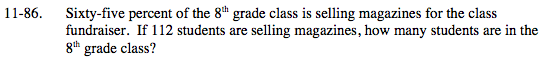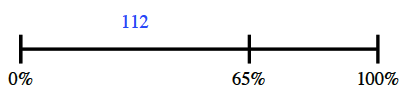### Home > MC2 > Chapter 11 > Lesson 11.3.1 > Problem11-86

11-86.112 students is 65% of the class.
Draw a diagram.

Now create a proportion equation that describes this relationship.

Approximately 172 students are in the 8th grade class.$\frac{65}{100}=\frac{112}x$